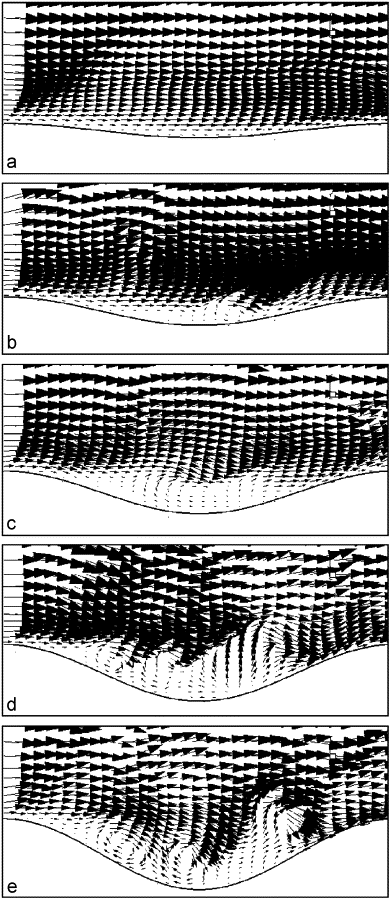# DIGITAL IMAGE

May 29 • Notes • 8045 Views • 1 Comment on DIGITAL IMAGE

DIGITAL IMAGE

DEFINATION:

IMAGE:-

• An  image is a 2D light intensity function f(X, Y) denotes spatial coordinates and which is at any point (X, Y) is proportional to the gray levels and brightness of the image at that point.
• Digital image has been discretized both in brightness and in spatial coordinates.
• Pixels are the element of such digital array and it is also called image elements.Digital image

Simplified diagram of a cross section of human eye

APPLICATION OF IMAGE PROCESSING

There are two application for digital image processing

1. For the human interpretation improvement of pictorial information.
2. For autonomous machine perception processing of scene data.

Example of digital image processing are military recognizance, automatic processing of finger print, automatic character recognition etc.

ELEMENTS OF DIGITAL IMAGE PROCESSING :

1. Acquisition
2. Storage
3. Processing
4. Communication
5. Display

SIMPLE IMAGE MODEL:

• An image f(x, y) must be digitalized both spatially and in amplitude for suitable computer processing.
• Image sampling is digitization of the spatial coordinates(x, y).
• And a gray-level quantization is amplitude digitization.
• As the number of gray-levels and spatial resolution increases the storage and processing requirement also increases.
• Example- A 256 gray-level image of size 256×256 occupies 64k bytes memory.
• There is a effect known as checkerboard effect caused when spatial resolution of images are very low
• False contouring is resulting due to insufficient number of gray-levels in the smooth areas .Digital image

Image of different amplitude resolution.

SAMPLING:

DEFINATION:

It is a process of conversion of continuous signal to discrete signal. For e.g.- conversion of a continuous signal sound wave into a sequence of sample which is a discrete time signal.

A value or set of values at a point in space or in time is refer as SAMPLE

Extraction of sample from a continuous signal is known as SAMPLER. Sampling is done for the function varying in time, space or any dimension.

Number of the sample obtained in one second is known as SAMPLING FREQUENCY or SAMPLING RATE. And it is denoted by   fs.

fs= 1/T

By the operation of sampling, quantizing and encoding digital signal is formed from the analog signal.

Analog signal is denoted by x(t) which is continuous in both amplitude and time. And after the sampling operation result is that the signal is continuous in amplitude but discrete in time. By encoding the time sampled value onto a finite set of value a digital signal is formed from a sampled data signal.

Spatial resolution of the digitized image is the sampling rate of the digitizer.

QUANTIZATION:

DEFINATION:

By compressing a range of values to a single quantum value QUANTIZATION for image processing is achieved. It is a lossy compression technique.

Quantization is a transition between continuous value of image function and its digital equivalent.

IMAGE QUANTIZATION

For enough human perception the number of quantization levels should be high. Image quantized with in sufficient brightness levels cause the false contour which is the main problem in the image. False contour occurs when the brightness level is lower than which human can easily distinguish.

QUESTION & ANSWER

1. What is a image??

ANSWER

An image is a 2D light  intensity function f(x, y), where (x, y) is the spatial coordinate and it is proportional to the gray-levels and the brightness of the image.

Image that has been discretized both in brightness and spatial coordinate is known as digital image.

Pixels are the element of such digital array.

2. What are the fundamental steps in digital image processing??

ANSWER

Steps in digital image processing are:

1. Image acquisition
2. Image processing
3. Image segmentation
4. Image description
5. Image recognition
6. Image interpretation

3.  What is a sampling??

Answer

It is a process of conversion of continuous signal into discrete signal. For e.g. conversion of continuous signal sound wave into sequence of sample which is discrete time signal.

4. What is quantization??

ANSWER

Quantization is a transition between continuous value of image function and its digital equivallent

### One Response to DIGITAL IMAGE

1. Shilpa Ranjan says:

Digital image is the most important part of digital system.Without digital image we can even imagine digital system and related technologies. The Article is an introduction on the topic digital image with some of the commonly asked questions asked with proper answer.Going through post can be informative and easy to understand..!!!!